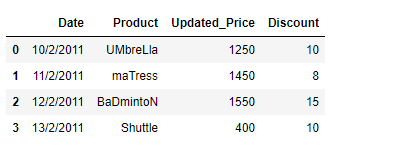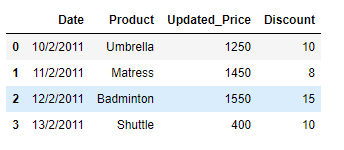# Clean the string data in the given Pandas Dataframe

As we know, In today’s world data analytics is being used by all sorts of companies out there. While working with data, we can come across any sort of problem which requires an out of the box approach for evaluation. Most of the Data in real life contains the name of entities or other nouns. It might be possible that the names are not in proper format. In this post, we are going to discuss the approaches to clean such data.

Suppose we are dealing with the data of an e-commerce based website. The name of the products is not in the proper format. Properly format the data such that the there are no leading and trailing whitespaces as well as the first letters of all products are capital letter.

Solution #1: Many times we will come across a situation where we are required to write our own customized function suited for the task at hand.

 `# importing pandas as pd ` `import` `pandas as pd ` ` `  `# Create the dataframe ` `df ``=` `pd.DataFrame({``'Date'``:[``'10/2/2011'``, ``'11/2/2011'``, ``'12/2/2011'``, ``'13/2/2011'``], ` `                   ``'Product'``:[``' UMbreLla'``, ``'  maTress'``, ``'BaDmintoN '``, ``'Shuttle'``], ` `                   ``'Updated_Price'``:[``1250``, ``1450``, ``1550``, ``400``], ` `                   ``'Discount'``:[``10``, ``8``, ``15``, ``10``]}) ` ` `  `# Print the dataframe ` `print``(df) `

Output :Now we will writer our own customized function to solve this problem.

 `def` `Format_data(df): ` `    ``# iterate over all the rows ` `    ``for` `i ``in` `range``(df.shape[``0``]): ` ` `  `        ``# reassign the values to the product column ` `        ``# we first strip the whitespaces using strip() function ` `        ``# then we capitalize the first letter using capitalize() function ` `        ``df.iat[i, ``1``]``=` `df.iat[i, ``1``].strip().capitalize() ` ` `  `# Let's call the function ` `Format_data(df) ` ` `  `# Print the Dataframe ` `print``(df) `

Output :Solution #2 : Now we will see a better and efficient approach using Pandas `DataFrame.apply()` function.

 `# importing pandas as pd ` `import` `pandas as pd ` ` `  `# Create the dataframe ` `df ``=` `pd.DataFrame({'``'Date'``:[``'10/2/2011'``, ``'11/2/2011'``, ``'12/2/2011'``, ``'13/2/2011'``], ` `                   ``'Product'``:[``' UMbreLla'``, ``'  maTress'``, ``'BaDmintoN '``, ``'Shuttle'``], ` `                   ``'Updated_Price'``:[``1250``, ``1450``, ``1550``, ``400``], ` `                   ``'Discount'``:[``10``, ``8``, ``15``, ``10``]}) ` ` `  `# Print the dataframe ` `print``(df) `

Output :Let’s use the Pandas `DataFrame.apply()` function to format the Product names in the right format. Inside the Pandas `DataFrame.apply()` function we will use lambda function.

 `# Using the df.apply() function on product column ` `df[``'Product'``] ``=` `df[``'Product'``].``apply``(``lambda` `x : x.strip().capitalize()) ` ` `  `# Print the Dataframe ` `print``(df) `

Output :My Personal Notes arrow_drop_upCheck out this Author's contributed articles.

If you like GeeksforGeeks and would like to contribute, you can also write an article using contribute.geeksforgeeks.org or mail your article to contribute@geeksforgeeks.org. See your article appearing on the GeeksforGeeks main page and help other Geeks.

Please Improve this article if you find anything incorrect by clicking on the "Improve Article" button below.

Article Tags :

Be the First to upvote.

Please write to us at contribute@geeksforgeeks.org to report any issue with the above content.